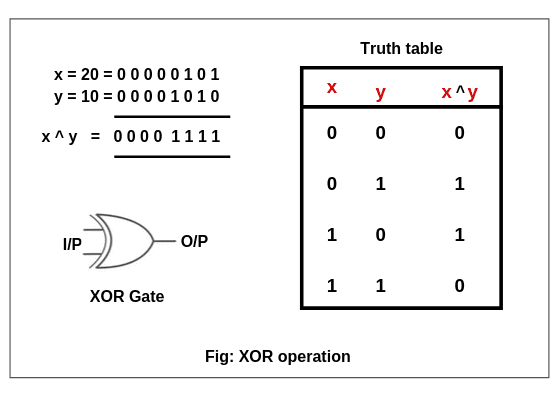# Bitwise Operator in Java | AND, OR, XOR

An operator that acts on individual bits (0 or 1) of the operands is called bitwise operator in Java.

It acts only integer data types such as byte, short, int, and long. Bitwise operators in java cannot be applied to float and double data types.

The internal representation of numbers in the case of bitwise operators is represented by the binary number system. Binary number is represented by two digits 0 or 1.

Therefore, these operators are mainly used to modify bit patterns (binary representation).

## Types of Bitwise Operators in Java

In Java, there are seven types of bitwise operators. They are listed in the below table form.

Table: Bitwise Operators

OperatorMeaning
1. &bitwise AND (Binary)
2. |bitwise OR (Binary)
3. ^bitwise exclusive OR (Binary)
4. ~bitwise NOT (Unary)
5. <<shift left
6. >>shift right
7. >>>unsigned right shift

We can also see the classification of bitwise operators in java in the below figure.## Bitwise AND Operator (&) in Java

This operator is used to perform bitwise AND operation between two integral operands. The AND operator is represented by a symbol & which is called ampersand. It compares each bit of the left operand with the corresponding bit of right operand.

Let’s consider the truth table given in the figure to understand the operation of AND operator.Truth table is a table that gives the relationship between inputs and output. In AND operation, on multiplying two or more input bits, we get output bit.

From the truth table shown in figure, if both compared input bits are 1, we get output 1. Otherwise, we get output 0.

From the truth table, On multiplying the individual bits of x and y, we get x & y = 0 0 0 0 1 0 1 0. It is nothing but 10 in decimal form. Let’s take an example program based on it.

Program code 1:

```package javaProgram;
public class BitwiseANDExample {
public static void main(String[] args)
{
int a = 10, b = 11;
System.out.println("(10 & 11): " +(a & b));
}
}
```
```Output:
(10 & 11): 10
```

## Bitwise OR Operator ( | ) in Java

This operator is used to perform OR operation on bits of numbers. It is represented by a symbol | called pipe symbol.

In OR operation, each bit of first operand (number)  is compared with the corresponding bit of the second operand.

Let us consider the truth table of the OR operator to understand OR operation.To understand OR operation, consider truth table given in the above figure. If both compared bits are 0, the output is 0. If any one bit is 1 in both bits, the output is 1.

On adding input bits of (x = 20) and (y = 10), we get output bits 0 0 0 0 1 1 1 1. This is nothing but 30 in decimal form.

Program code 2:

```package javaProgram;
public class BitwiseORExample {
public static void main(String[] args)
{
int a = 20, b = 10;
System.out.println("(20 | 10): " +(a | b));
}
}
```
```Output:
(20 | 10): 30
```

## Bitwise Exclusive OR (XOR) Operator (^)

The operator ^ performs exclusive OR (XOR) operation on the bits of numbers. This operator compares each bit first operand (number) with the corresponding bit of the second operand.

Exclusive OR operator is represented by a symbol ^ called cap. Let us consider the truth table of the XOR operator to understand exclusive OR operation.To understand exclusive OR (XOR) operation, consider the truth table as shown in the above figure. If we have odd number of 1’s in input bits, we get output bit as 1.

In other words, if two bits have the same value, the output is 0, otherwise the output is 1.

From the truth table, when we get odd number of 1’s then notice that the output is 1 otherwise the output is 0. Hence, x ^ y = 0 0 0 0 1 1 1 1 is nothing but 30 in decimal form.

Program code 3:

```package javaProgram;
public class BitwiseXORExample {
public static void main(String[] args)
{
int a = 20, b = 10;
System.out.println("(20 ^ 10): " +(a ^ b));
}
}
```
```Output:
(20 ^ 10): 30
```

## Bitwise NOT operator (~)

The bitwise NOT operator in Java is basically an inverter. It returns the reverse of its operand or value. It converts all 1s to 0s, and all the 0s to 1s. Therefore, it is also called unary operator or bit flip or one’s complement operator.

The truth table of bitwise NOT operator is as below:

A~A
01
10

Let’s take a simple example program, where we will perform all operations based on bitwise operators in Java.

Program code 4:

```package javaProgram;
public class BitwiseOperatorsEx {
public static void main(String[] args)
{
int a = 2, b = 10;
System.out.println("(2 & 10): " +(a & b));
System.out.println("(2 | 10): " +(a | b));
System.out.println("(2 ^ 10): " +(a ^ b));
System.out.println("~10: " +~b);
}
}
```
```Output:
(2 & 10): 2
(2 | 10): 10
(2 ^ 10): 8
~10: -11
```

In this tutorial, you learned types of bitwise operators in Java with example programs. Hope that you will have understood all the basic points related to bitwise AND, OR, XOR, and NOT operators. In the next, we will discuss shift operators in Java with various example programs.